# Fermat's little theorem

## Statement

### For a relatively prime number

Supposeis a prime number andis a natural number that is not a multiple of. Then, the following equivalent statements hold:

1. (Divisibility form):divides.
2. (Congruence form):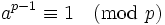.
3. (Order of element modulo another): The order ofmodulodivides.

### For a not necessarily relatively prime number

Supposeis a prime number andis a natural number, not necessarily relatively prime to. Then, the following equivalent statements hold:

1. (Divisibility form):divides.
2. (Congruence form):.

## Related facts

### Stronger facts

• Euler's theorem: This is a general version which states that ifandare relatively prime, then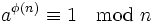whereis the Euler phi-function of, i.e., the number of natural numbers less than or equal tothat are relatively prime to. The Euler ph-i-function of a primeis, so this is a generalization.
• Order of element divides order of group: In a finite group, the order of any element divides the order of the group. This is a corollary of Lagrange's theorem in group theory. Fermat's little theorem is a special case of this where the group is the multiplicative group modulo, which has order.
• Primitive roots exist modulo primes: For any prime, there exists a natural numberrelatively prime tosuch thatis precisely equal to the order ofmod: in other words, no smaller power ofismodulo. Such anis termed a primitive root. This follows from the fact that the multiplicative group of a prime field is cyclic, which in turn follows from the more general fact that multiplicative group of a field implies every finite subgroup is cyclic.

### Other related facts

• Wilson's theorem: This states that for any prime,.
• For a givenmodulothat is relatively prime to,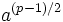ismoduloif and only ifis a quadratic residue modulo.
• Period in decimal expansion of reciprocal of prime divides prime minus one: Suppose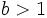is chosen as a base for writing numbers, andis a prime that does not divide. Then, the expansion ofin basehas period dividing. In fact, the period equals the order ofmodulo, and it dividesby Fermat's little theorem. It equalsif and only ifis a primitive root modulo. For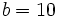, such primesare termed full reptend primes.

### Converse

If, for a givenrelatively prime to,divides, it does not follow thatis prime. This leads to some terminology:

• Fermat pseudoprime to baseis a composite numbersuch thatandare relatively prime anddivides. (Caution: Fermat prime means something very different!)
• Poulet number is a Fermat pseudoprime to base.
• Carmichael number, also called absolute pseudoprime, is a composite natural numbersuch thatdividesfor allrelatively prime to. Equivalently,divides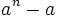for all.